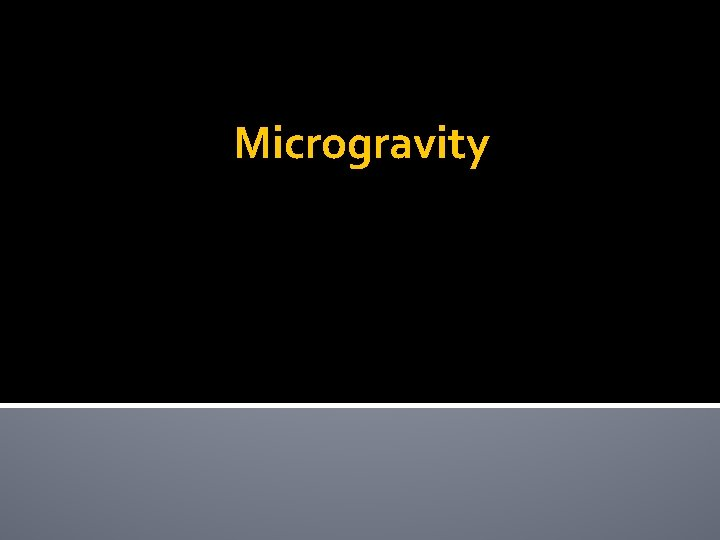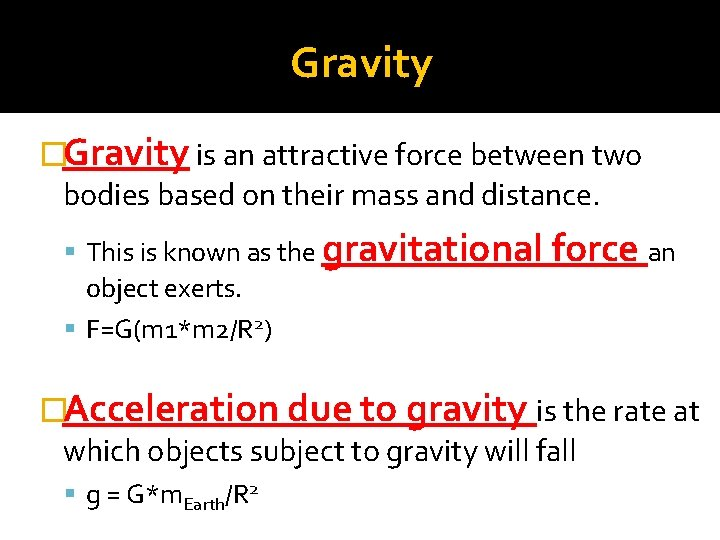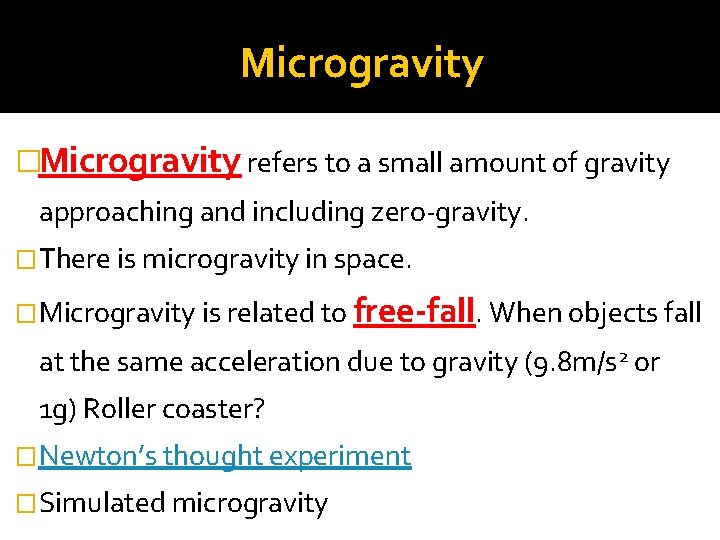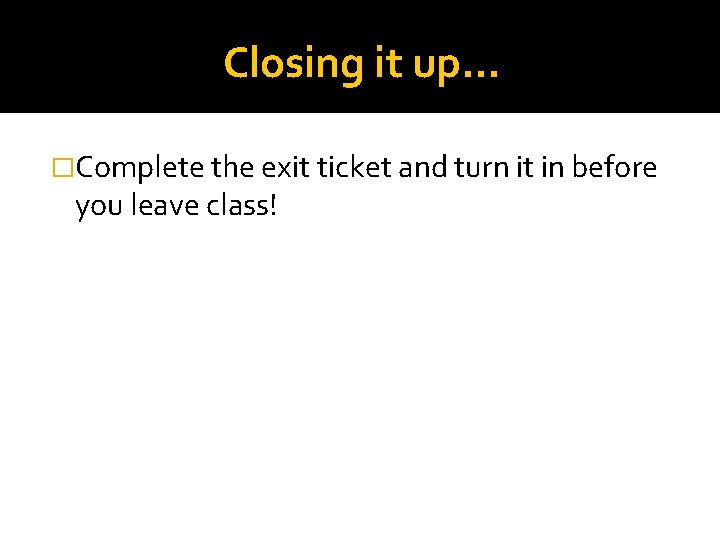# Microgravity Gravity Gravity is an attractive force between

• Slides: 7MicrogravityGravity �Gravity is an attractive force between two bodies based on their mass and distance. This is known as the gravitational object exerts. F=G(m 1*m 2/R 2) force an �Acceleration due to gravity is the rate at which objects subject to gravity will fall g = G*m. Earth/R 2Microgravity �Microgravity refers to a small amount of gravity approaching and including zero-gravity. �There is microgravity in space. �Microgravity is related to free-fall. When objects fall at the same acceleration due to gravity (9. 8 m/s 2 or 1 g) Roller coaster? �Newton’s thought experiment �Simulated microgravityInverse Square Law �Formula Fgrav ~ 1/d 2 Where d = distance in meters Since distance is raised to the 2 nd power, it is said that the force of gravity is inversely related to the square of the distance. If Fgrav goes up, then distance goes down. If distance goes up, then Fgrav goes down. Because distance is squared, changes in distance have a large affect.Let’s Break it Down! �Take out your calculators. �Complete the table on your worksheet to see how much gravity is reduced as distance increases or decreases!Let’s Practice! �Work alone or in pairs to complete the questions on your worksheet. �Review as a class.Closing it up… �Complete the exit ticket and turn it in before you leave class!